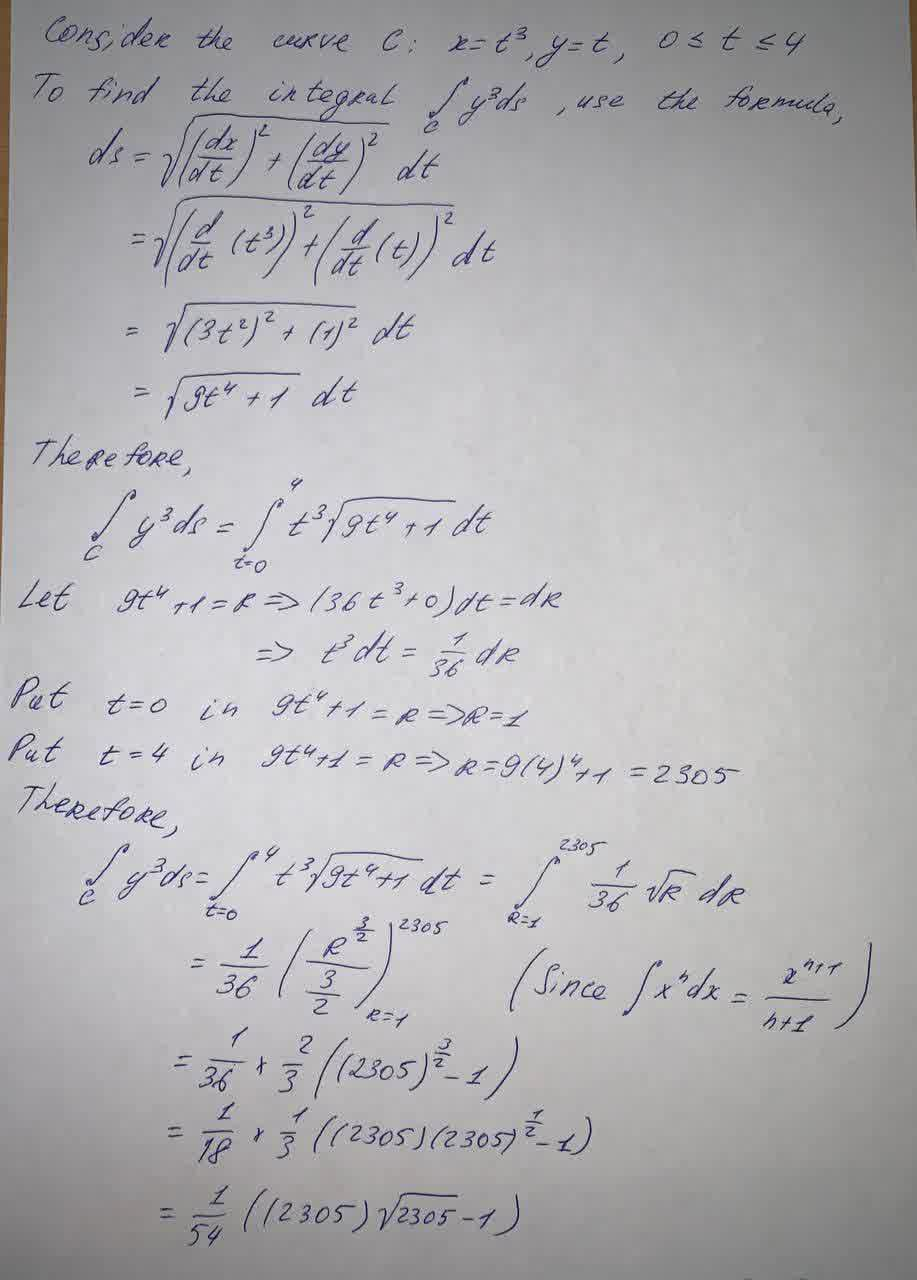Question# Evaluate the line integral, where C is the given curve. C y^3 ds, C: x = t^3, y = t, 0 \leq t \leq 4

Integrals
ANSWEREDEvaluate the line integral, where C is the given curve. C $$y^3 ds, C: x = t^3, y = t, 0 \leq t \leq 4$$